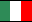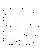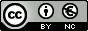South France in sequence

T r a v e l s | K u r d i s t a n | I t a l i a n P i c t u r e s
B a n k n o t e s | G r a f f i t i & M u r a l s | L i n k s | I n t r o d u c t i o n
H O M E P A G E
I T A L I A N H O M E P A G Eitaliano